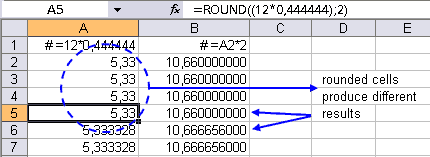## Round up formulas with =ROUND()

I just got a call from a friend of mine. He needed to edit a spreadsheet and round a bunch of formulas. Since the rounding is important for the calculations, a simple cell-formatting wouldn't do. Excel's worksheet function =ROUND() can be used to round the numbers. However editing a lot of cells by hand (especially with a lot of different formulas that can't be copied) can be time consuming. So I wrote a little routine for it he can use the next time:```Sub BuildRoundFormula()
' Build the =ROUND() function around the formulas

Dim rngCel             As Range
Dim strFormula         As String
Dim lNumDigits         As Long
lNumDigits = 2            ' < --- EDIT THIS

' If lNumDigits is greater than 0 (zero), then number
' is rounded to the specified number of decimal places.
' If lNumDigits is 0, then number is rounded to the
' nearest integer.
' If lNumDigits is less than 0, then number is rounded
' to the left of the decimal point.

For Each rngCel In Selection
If rngCel.HasFormula Then
strFormula = rngCel.Formula
' strip the equal sign on the left:
strFormula = Right(strFormula, Len(strFormula) - 1)
rngCel.Formula = "=ROUND((" & strFormula & ")," & _
lNumDigits & ")"
End If
Next
End Sub```
By changing the value of the variable lNumDigits you can specify the number of decimals or digits. If you're a little handy with macro's you can use this procedure as a start to apply other functions.

#### Bastien

Website: https://www.asap-utilities.com##### Jon Peltier

I've seen this done in the case when a set of formulas frequently returns errors. The code is designed to turn this:

=SomeFunction

into this:

=IF(ISERR(SomeFunction),ErrorValue,SomeFunction)##### Bastien

Hello Jon,

I've recently written a short post on formula error handling. That post was more aimed on people that know little to nothing about macros and shows how ASAP Utilities comes in handy.
//www.asap-utilities.com/blog/index.php/2005/10/07/hide-formula-errors-like-na-or-value/